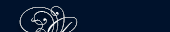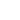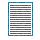НОВОСТИ   БИБЛИОТЕКА   ЭНЦИКЛОПЕДИЯ   КАРТА САЙТА   ССЫЛКИ   О САЙТЕ## THE HISTORY OF GEOMETRY

Geometry is the Greek name for the science which the early Egyptians began and developed about 5000 years ago. The word geometry is derived from two Greek words: geo meaning earth and metron meaning measure.

For erecting pyramids the early Egyptians needed professional geometers who were able to locate a line running north .and south.

The geometry known to the Egyptians consisted principally of rules and formulas for finding areas and volumes. The Egyptians were principally interested in the practical application of their rules.

After a time Greek philosophers and teachers developed and perfected the proofs of the Egyptians. The most important of the early Greek teachers was Pythagoras who was born about 569 before our era. He founded a school in Italy. The students were divided into two classes - beginners and Pythagorians.

Plato, who lived more than a hundred years later than Pythagoras, was primarily a philosopher. His interest in geometry was not because of its practical use, but because of the logic contained in the proofs.

The best known name in connection with geometry is Euclid. Euclid was a teacher of geometry in Alexandria. He used to say that geometry trained the habits of expressing thoughts accurately. One of his most important textbooks is called The Elements. The Elements of Euclid has been used as a basis for all textbooks on geometry since his time.

Another famous scientist of ancient, times was Archimedes who lived in Sicily. Archimedes discovered many laws of mathematics.

For over twenty centuries Euclidean geometry was the ruling theory. In the 19th century the Russian mathematician Lobatchevsky founded non-Euclidean geometry of two dimensions. Such kind of geometry is called hyperbolic. It is based on the assumption that the axiom on parallels is not true, and through a point any number of straight lines can be drawn parallel to a given straight line.

The third system of geometry was developed by Riemann and is called elliptic geometry. Riemann assumes that no straight line can be drawn which will not meet any other straight line.

Thus we have three systems of geometry.

assumption предположение

axiom parallels аксиома, параллелей

Euclid Евклид

Plato Платон

Pythagoras Пифагор

Sicily СицилияПОИСК: